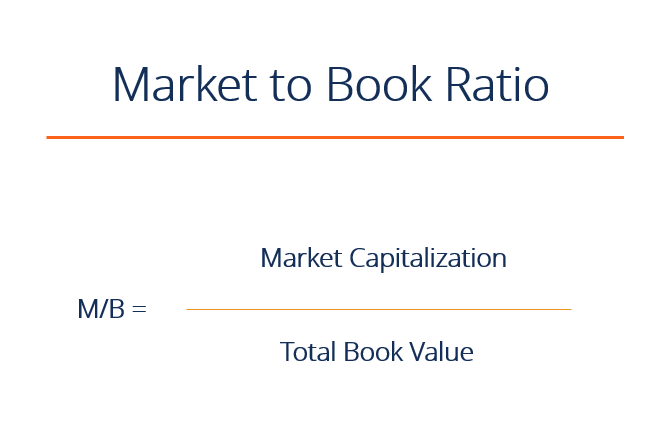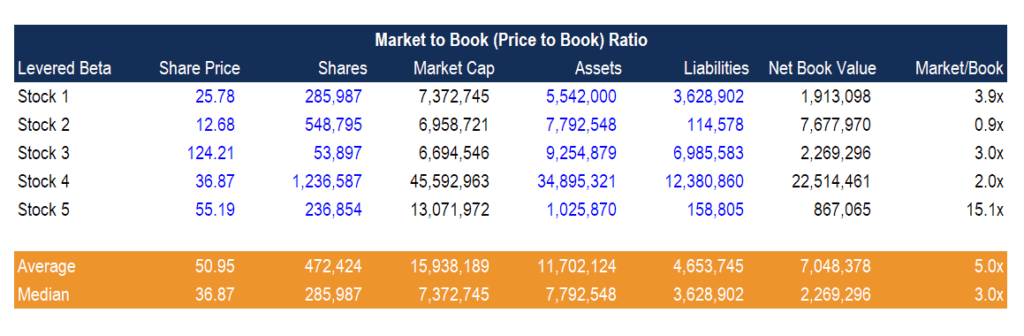Market to Book Ratio

Explanation of the market to book ratio, how to calculate it, and what it's used for

What is the Market to Book Ratio (Price to Book)?

The Market to Book ratio (also called the Price to Book ratio), is a financial valuation metric used to evaluate a company’s current market value relative to its book value. The market value is the current stock price of all outstanding shares (i.e. the price that the market believes the company is worth). The book value is the amount that would be left if the company liquidated all of its assets and repaid all of its liabilities. The book value equals the net assets of the company and comes from the balance sheet. In other words, the ratio is used to compare a business’s net assets that are available in relation to the sales price of its stock.The market to book ratio is typically used by investors to show the market’s perception of a particular stock’s value. It is used to value insurance and financial companies, real estate companies, and investment trusts. It does not work well for companies with mostly intangible assets. This ratio is used to denote how much equity investors are paying for each dollar in net assets.

The market-to-book ratio is calculated by dividing the current closing price of the stock by the most current quarter’s book value per share.

Market to Book Ratio Formula

The Market to Book formula is:

Market Capitalization / Net Book Value

or

Share Price /  Net Book Value per Share

Where, Net Book Value = Total Assets – Total Liabilities

Interpreting the Ratio

A low ratio (less than 1) could indicate that the stock is undervalued (i.e. a bad investment), and a higher ratio (greater than 1) could mean the stock is overvalued (i.e. it has performed well). Many argue the opposite and due to the discrepancy of opinions, the use of other stock valuation methods either in addition to or instead of Price to Book ratio could be beneficial for a company.

A low ratio could also indicate that there is something wrong with the company. This ration can also give the impression that you are paying too much for what would be left if the company went bankrupt.

The market-to-book ratio helps a company determine whether or not its asset value is comparable to the market price of its stock. It is best to compare Market to Book ratios between companies within the same industry.

Example Calculation of Price to Book Ratio in Excel

The Price to Book ratio (or Market to Book ratio) can easily be calculated in Excel if the following criteria are known: share price, number of shares outstanding, total assets, and total liabilities.  From there, market capitalization and net book value can be calculated. Market Cap is equal to share price times shares outstanding. Net Book Value is equal to Total Assets minus Total Liabilities.As you can see in the example above, all assumptions or hardcodes are in blue font, and all formulas are in black.

Stock 1 has a high market capitalization relative to its net book value of assets, so its Price to Book ratio is 3.9x.

Stock 2 has a lower market cap than it’s book value of equity, so its Market to Book ratio is 0.9x.

Enter your name and email in the form below and download the free template now!

How is the Market to Book Formula Derived?

The Market to Book multiple can be shown to be equal to PE x ROE by doing some financial analysis.  It is therefore driven by return on equity and the drivers of the PE multiple.

It can also be shown that the PE multiple is driven by (1 – g/ROE) / (r – g) where r is the cost of equity, g is the growth rate, and ROE is return on equity.

Since the MB multiple is PE x ROE, this means the MB multiple is (ROE – g) / (r – g).  If we assume a zero growth rate, the equation implies that the market value of equity should be equal to the book value of equity if ROE = r.

The MB multiple will be higher than 1 if a company delivers ROE higher than the cost of equity (r).

This has been a guide to the market to book ratio and formula. To keep learning and advancing your career we highly recommend these additional free resources:

• Analysis of financial statements
• Valuation ratios
• Comparable company analysis
• Financial modeling guide

Valuation Techniques

Learn the most important valuation techniques in CFI’s Business Valuation course!

Step by step instruction on how the professionals on Wall Street value a company.

Learn valuation the easy way with templates and step by step instruction!